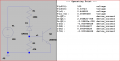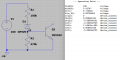# What is my voltage at the two question marks?

Joined Nov 28, 2021
13
say i have 100v input to this circuit, what would my voltage be at each of my question marks? and could you explain the math behind it? trying to learn circuitry without any formal schooling. this one has me stumped due to the zener since apparently it shunts the differential voltage to the components downstream? any help is appreciated.
also that should be 470k ohms btw.

Last edited:

#### dl324

Joined Mar 30, 2015
15,838
Which terminal is the collector on the transistor?

Is this school work?

#### MrChips

Joined Oct 2, 2009
29,241
Remove the transistor for now.
Assume the current = 95V / 570kΩ = 167μA

#### ErnieM

Joined Apr 24, 2011
8,362
Your schematic is not completely clear so I had to make a few assumptions:

- The 100V is connected between "Voltage In" and the ground symbol.

- The transistor base is connected to the 470K resistor

- The transistor Emitter is connected to ground.

Even if you were to check the data sheet for the 2N5551 you would not find any info about the base to emitter drop, so you would need to either measure it in circuit, or use a "good guess." A typical B-E drop is around 0.7V so let's use that.

OK, the first voltage? is at ground, so that voltage IS ground, or zero volts.

The next voltage is at the junction of the zener, 470K, and the base. From the above info we see the emitter is ground (0V) and the base is 0.7 volts above that, so voltage? there is 0.7-0=0.7 volts.

Now let's look a little deeper. The voltage between the zener and the 100K is 5.0V greater than the base, or 5.7V, so the 100K sees 100-5.7=94.3V. Thus the current thru the 100K is 94.3/100K=0.943mA.

Back at the base the 470K will sink 0.7V/470K=1.49uA (micro amps). That current comes from the 100K/zener portion leaving the remainder for the base. Thus the base gets .943mA-1.49uA=.942mA

Note: removing the transistor for analysis yields nothing useful.

#### KeithWalker

Joined Jul 10, 2017
2,872
Actually, removing the transistor is essential for the initial analysis. If, with the transistor removed, The voltage is less than 0.7V, then it will be approximately the same with the transistor in circuit

#### Irving

Joined Jan 30, 2016
3,496
@ErnieM I think you'll find the lower resistor is 470ohm not 470k! And taking the transistor out of circuit is an essential step.

@thunderhead289 Taking the transistor out of circuit, you can apply Kirchoff's current law to the circuit. Assuming the zener diode is operating, the total voltage across the 2 resistors is 100-Vz = 95v, therefore the current through both of them is 95/(100k+470) = 0.9456mA, and therefore the voltage at the top of the 470ohm is 470 x 0.9456 mV = 444mV. Since this is < the typical Vbe of 0.7v for a silicon NPN we can assume the transistor has little or no effect at 100v - that may not be true at a higher voltage.

A circuit simulator is useful to check calculations (some might argue that knowing how to do the calculations is important to check the simulation!).

Here is your circuit in LTSpice doing a DC operating point calculation: as you can see the current in R2 is 0.9466mA, very close to the calculation above. There is a tiny base current as the transistor base-emitter junction isn't a perfect diode, but at 20nA. we were right to ignore it.Last edited:

#### Audioguru again

Joined Oct 21, 2019
6,162
You do not show if the 100V input voltage is positive or negative.
You do not show which way the transistor is facing.
Here are its pins from its datasheet:

#### ErnieM

Joined Apr 24, 2011
8,362
@ErnieM I think you'll find the lower resistor is 470ohm not 470k!
@ErnieM And taking the transistor out of circuit is an essential step.
I think not as the BE junction limits the voltage across the 470K resistor.

#### Irving

Joined Jan 30, 2016
3,496
OK, I stand corrected and I concur with your analysis. However, I'd still argue you need to take the transistor out of circuit to validate that the voltage at v1 is being clamped at Vbe. In this instance, with 470k, its a fair bet as simplistically 470/(470+100) * 95 = 78v, but with lower value resistors < 1k its not so obvious.

Its not immediately obvious what this circuit is intended to do - other than its a switch of some sort but the 5v zener is somewhat superfluous - it's not protecting the base-emitter junction against reverse breakdown if this were high-voltage AC for example.

For completeness here's the simulation...#### Audioguru again

Joined Oct 21, 2019
6,162
Without a detailed schematic, we do mot know how the circuit is.